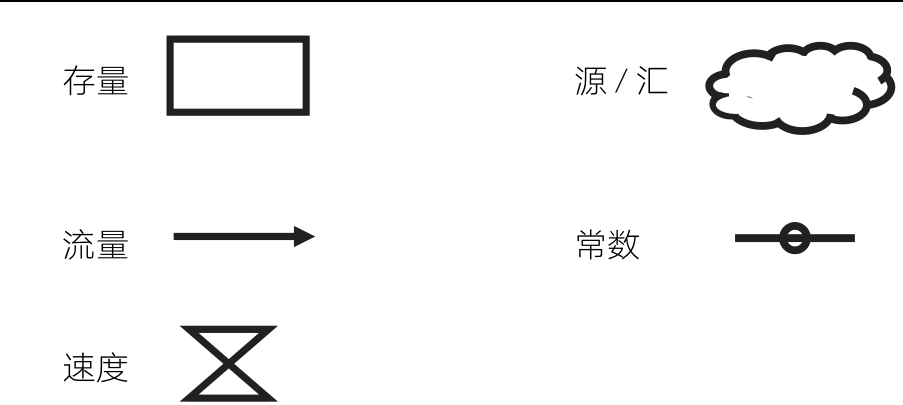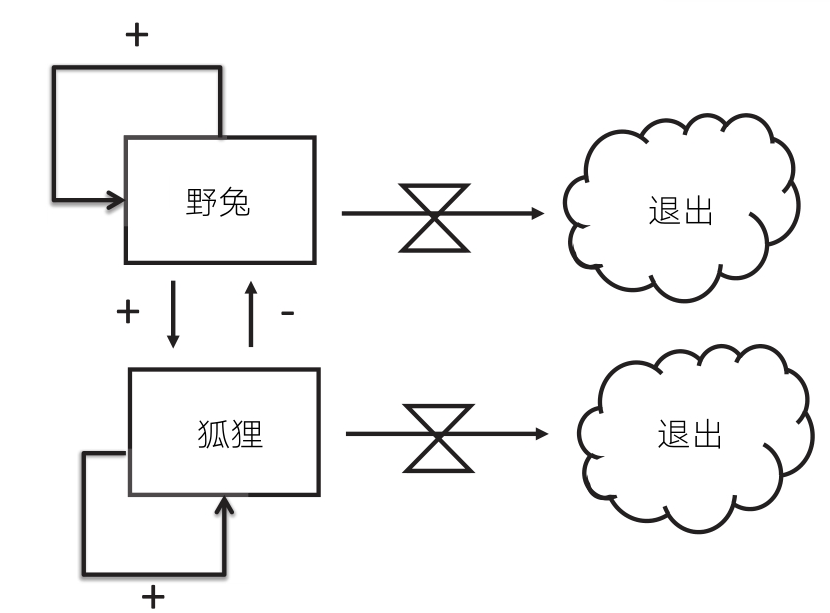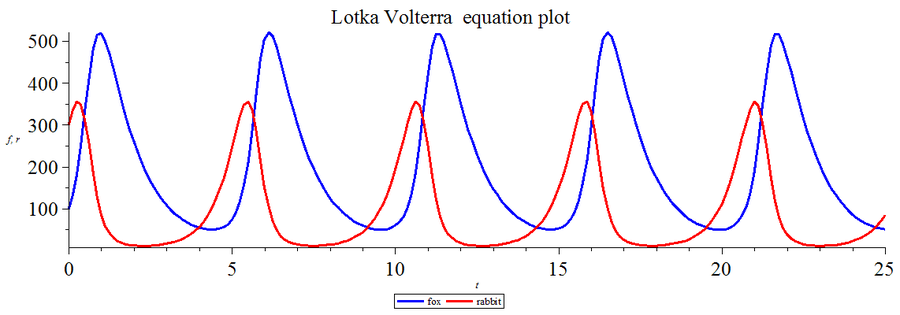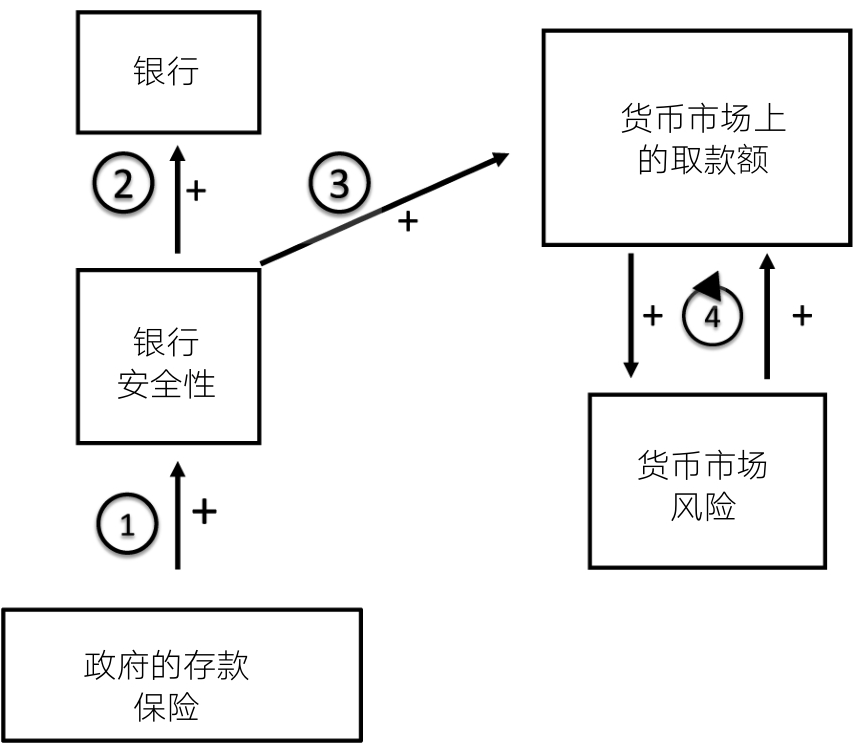## 基本概念## 捕食者-猎物模型$${\frac {dx}{dt}}=x(g -a y)$$ $${\frac {dy}{dt}}=y(b x -d)$$## 应用